﻿ Ground Water Prediction Using Bacterial Foraging Optimization Technique

### Ground Water Prediction Using Bacterial Foraging Optimization Technique

Dixit Prasanna Kumar, Sahoo Archana

Journal of Computer Sciences and Applications

## Ground Water Prediction Using Bacterial Foraging Optimization Technique

Dixit Prasanna Kumar1,, Sahoo Archana2

1Interface Bhubaneswar

2Chief Technology Architect, Interface Software, Bhubaneswar

### Abstract

Ground water in the water located beneath the earth’s surface in soil pore spaces and in the patterns of rock formations. The ways to determine the level of ground water is called ground water prediction. The ground water level is the important factors which affect the development of national economy and society. It is needed to predict ground water because in recent times too much water is pumped out from under ground . In the State of Odisha (India), more than 85% of geographical area falls under consolidation formations with low groundwater development status. In places like Puri, Bhubaneswar ground water can be found at around 200ft down but in case of rocky areas like Keonjhar, western Odisha such as Koraput, Titlagarh etc., it is very hard to reach the ground water level which is very deep down the earth surface. Another problem is the authorities from water resource development use to dig unnecessary pot holes in search of ground water without having any information about the availability of water. These pot holes create problems for the local people. It leads to wastage of both time and money of government. So this paper proposes a method through this we can overcome the above problem. In this paper the availability of ground water was predicted by using some prediction techniques like linear regression, multi-linear regression. Regression analysis can be used to model the relationship between one or more independent or predictor variables and a dependent or response variable (which is continuous-valued). In other words, a regression model is a mathematical model that predicts a continuous response variable. And also we are introducing the prediction of ground water by using a nature inspired Bacterial Foraging Optimization (BFO). This technique is proposed by K.M. Passino in 2002 to handle complex problems of the real world . Bacterial Foraging Optimization Algorithm (BFO) is inspired by the foraging and chemotactic phenomenon of bacteria. Bacterial Foraging Optimization (BFO) algorithm is a new class of biologically stochastic global search technique based on the foraging behaviour of E. coli bacteria. This method is used for locating, handling, and ingesting the food. During foraging, a bacterium can exhibit two different actions: tumbling or swimming which can be simulated for predicting the ground level water.

• Dixit Prasanna Kumar, Sahoo Archana. Ground Water Prediction Using Bacterial Foraging Optimization Technique. Journal of Computer Sciences and Applications. Vol. 3, No. 6, 2015, pp 172-176. http://pubs.sciepub.com/jcsa/3/6/12
• Kumar, Dixit Prasanna, and Sahoo Archana. "Ground Water Prediction Using Bacterial Foraging Optimization Technique." Journal of Computer Sciences and Applications 3.6 (2015): 172-176.
• Kumar, D. P. , & Archana, S. (2015). Ground Water Prediction Using Bacterial Foraging Optimization Technique. Journal of Computer Sciences and Applications, 3(6), 172-176.
• Kumar, Dixit Prasanna, and Sahoo Archana. "Ground Water Prediction Using Bacterial Foraging Optimization Technique." Journal of Computer Sciences and Applications 3, no. 6 (2015): 172-176.

 Import into BibTeX Import into EndNote Import into RefMan Import into RefWorks

1
Prev Next

### 1. Introduction

The top layer of earth is dirt, but as we get deeper, the dirt changes into layers of solid rock. Each of these layers has many small spaces and cracks filled with water. Ground water is the water that is found beneath the earth's surface in the patterns of rock formations. Groundwater has an important role in the environment: it replenishes streams, rivers, and wetlands and helps to support wildlife habitat; it is used as primary source of drinking water and also in agricultural and industrial activities. Regression analysis can be used to model the relationship between one or more independent or predictor variables and a dependent or response variable (which is continuous-valued). In other words, a regression model is a mathematical model that predicts a continuous response variable. For example, a regression model could be developed to predict actual sales volume or the temperature resulting from an experiment. Bacterial foraging optimization is first proposed by Passino in 2002. This algorithm is inspired by the foraging and chemotactic behavior of bacteria Escherichia Coli (E. coli). The process of searching nutrients is known as foraging. The original Bacterial Foraging Optimization system consists of three principal mechanisms, namely, chemotaxis, reproduction, and elimination-dispersal. Chemotactic consists of Swim and Tumble.

### 2. Review of Literature

1. Chandni et. al. proposed linear regression and multiple-Linear regression are efficient prediction technique to predict ground water possibility and author has developed a system which can predict ground water level at any place without drilling holes by knowing its geological features only . The other possible applications may be processing medical data where integration of Bacteria Foraging and CBR system can be used to diagnose the disease of patients.

2. Ranu Rani Sethi et.al presented a procedure which determines the factors that influence and control the water table fluctuation in a specific geomorphologic situation. Author has developed a forecasting model and examines its potential in predicting water table depth using limited data and also developed different models for prediction of water table depth based on artificial neural networks (ANNs) .

3. Sara Maatta presents two models which predict groundwater levels in an unconfined shallow aquifer in the Sears Ville basin, part of the Jasper Ridge Biological Preserve. She took input data (i.e. features) for the models as local weather, lake stage, and stream flow data, and moving averages of the weather, stage and stream flow data taken over time-frames of one week, one month, three months and six months. The author conclude that linear regression does a good job of predicting groundwater levels in the summer, when water levels are low, while the neural network does a good job of predicting groundwater levels in the winter, when water levels are high .

4. MAO Xiaomin et.al. gave the idea that the prediction of groundwater level is important for the use and management of ground water resources. Author uses the artificial neural networks (ANN) to predict groundwater level in the Dawu Aquifer of Zibo in Eastern China . The author suggests that the first step was an auto-correlation analysis of the groundwater level which showed that the monthly groundwater level was time dependent.

5. Jianhua Tao et.al. developed a predicting method of ground water level based on Back Propagation neural network. His experimental results that show the predicting model has high precision. This paper is able to describe complicated system of ground water, and reveals nonlinear characteristics of ground water movement .

6. Ritanjali Majhi et.al. introduces the Bacterial Foraging Optimization(BFO) technique to develop an efficient forecasting model for prediction of various stock indices. Here she wants to show the difference between BFO and multilayer perceptr on base model in stock market prediction. Finally she conclude that BFO model is computationally more efficient, prediction wise more accurate and takes less training time compared to the standard MLP based model .

7. Dharminder Kumar et.al. proposed an optimization technique, Bacterial Foraging Optimization (BFO) to tackle complex search problems of the real world. It is also used to find the optimal solution of the problem. His research work suggests that images quantized with Bacteria Foraging Optimization technique gives good results . He explains the Bacteria Foraging Optimization for Colour Image Quantization and Presents the evaluation for various file formats.

8. Dr Shyam. S. Pattnaik et.al. developed a novel technique by hybridizing Bacterial Foraging Optimization technique in conjunction with Particle Swarm Optimization and is applied to the expressions developed by M.Kara to calculate accurately the desired resonant frequency of rectangular micro strip antenna of any dimension and of any substrate thickness . After his experiment, the result shows promising improvement in the accuracy, while achieving drastic reduction in computational time.

### 3. Preliminary Concepts

Prediction

Prediction is a form of data analysis that can be used to extract models describing important data classes or to predict future data trends. A predictive model will calculate an estimate for one or more variables (responses), based on other variables (descriptors).Prediction is used where an estimate or forecast is required, for example, to project sales or forecast the weather. Prediction is used to understand the relationship between the input descriptor variables and the output response variables . There are different types of prediction techniques that are used for prediction:

•  Regression Techniques

•  Numeric Prediction

Regression analysis can be used to model the relationship between one or more independent or predictor variables and a dependent or response variable (which is continuous-valued). In other words, a regression model is a mathematical model that predicts a continuous response variable. Regression is of three types:

a. Linear Regression

b. Non-Linear Regression

c. Multiple-Linear Regression

Linear Regression

•  Linear Regression analysis involves a response variable y, and a single predictor variable, x.

•  It is the simplest form of regression, and models y as a linear function of x.

That is,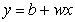(1)

Where,

~Variance of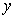is assumed to be constant.

~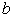and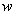are regression coefficients.

•  The regression coefficients, w and b, can also be thought of as weights, so that we can equivalently write,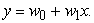(2)

•  Let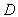be a training set consisting of values of predictor variable, x, for some population and their associated values for response variable, y.

•  The regression coefficients can be estimated using this method with the following equations: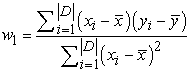(3)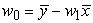(4)

Where,

~= Training Set.

~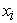= Total Number of predicted variables.

~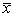= Mean value of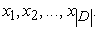~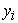= Total number of actual variables.

~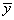= Mean value of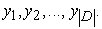Multiple-Linear Regression

•  Multiple-Linear regression is an extension of straight-line regression so as to involve more than one predictor variable.

•  It allows response variable y to be modelled as a linear function of, say, n predictor variables or attributes, A1, A2…, An, describing a tuple, X. (That is, X =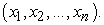)

•  Our training data set, D, contains data of the form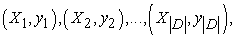where the Xi are the n-dimensional training tuples with associated class labels, yi.

•  An example of a multiple linear regression model based on two predictor attributes or variables, A1 and A2, is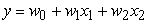(5)

Wherex1 and x2 are the values of attributes A1 and A2, respectively, in X. The method of least squares shown above can be extended to solve for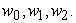Bacterial Foraging Optimization (BFO)

Bacterial foraging optimization is first proposed by Passino in 2002.This algorithm is inspired by the foraging and chemotactic behavior of bacteria Escherichia coli (E. coli). This optimization is used in many areas like: Ground-water prediction, Stock-market prediction, Weather prediction, Disease prediction etc .

CLASSICAL BFO ALGORITHM

The original Bacterial Foraging Optimization system consists of three principal mechanisms, namely, chemotaxis, reproduction, and elimination-dispersal. Chemotactic consists of Swim and Tumble. Each of these processes is described as follows.

Chemo taxis

In the original BFO, a unit walk with random direction represents a “tumble” and a unit walk with the same direction in the last step indicates a “run”. Suppose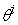(j,k,l) represents the bacterium at jth chemotactic, kth reproductive, and ith elimination-dispersal step. C(i) is the chemotactic step size during each run or tumble (i.e., run-length unit). Then in each computational chemotactic step, the movement of the ith bacterium can be represented as: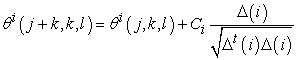Where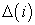is the direction vector of the jth chemotactic step. when the bacterial movement is run,is the same with the last chemotactic step; otherwise,is a random vector whose elements lie in [-1,1]. With the activity of run or tumble taken at each step of the chemotaxis process, a step fitness, denoted as J(i,j,k,l), will be evaluated.

Reproduction

The health status of each bacterium is calculated as the sum of the step fitness during its life, that is,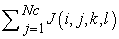， where Nc is the maximum step in a chemotaxis process. All bacteria are sorted in reverse order according to health status. In the reproduction step, only the first half of population survives and a surviving bacterium splits into two identical ones, which are then placed in the same locations. Thus, the population of bacteria keeps constant.

Elimination and Dispersal

The chemotaxis provides a basis for local search, and the reproduction process speeds up the convergence which has been simulated by the classical BFO. While to a large extent, only chemotaxis and reproduction are not enough for global optima searching. Since bacteria may get stuck around the initial position or local optima, it is possible for the diversity of BFO to change either gradually or suddenly to eliminate the accidents of being trapped into the local optima. In BFO, the dispersion even happens after a certain number of production processes. Then some bacteria are chosen, according to a preset probability Ped, to be killed and moved to another position within the environment.

The original BFO algorithm is briefly outlined step by step as follows.

ALGORITHM CBR Using BFO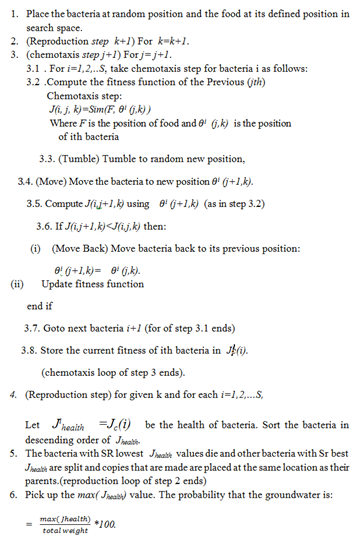4. Experimental Evaluation and Result Analysis

Dataset Used

All the details about the area, where we are going to predict the ground water posibility is collected. Details are followed by the attributes like Geology, Landform, Soil, Land use, Slope and Lineament. From those details a data set is prepared. The six attributes have their own value. The six attributes and its values are representing in the Table 1 given below:

Result Analysis

The predictions are done by using Linear Regression and Multiple Linear Regression technique. Then all the errors are calculated. After analysis it is found that Multiple Linear Regression is best prediction technique. Then an optimization technique is proposed to optimize the error values. After optimization it is found that BFO is best. The figure given below shows the errors in all the techniques. The errors are as follows, For place 6 error of BFO is 2.5,for Multiple Linear Regression the error is 3.0 and for Linear Regression it is 15.0 in Figure 1. Therefore it is analyzed that BFO has less error and hence BFO is best technique. Groundwater possibility is high with 81.5%.

#### Table 1. Six attributes of ground water possibility detectionDownload asPowerPoint Slide

Veiw figure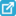View current table in a new windowDownload asVeiw figureFigures index
Figure 1. Error plot in different prediction techniques

### 5. Conclusion

In this paper, we have presented linear regression and Multiple Linear regression as an efficient prediction technique to predict ground water possibility in a given area. The results obtained in this work are comparable to the work already done in this field. A system has been developed which can predict ground water level at any place without drilling holes by knowing its geological features only. The system developed is of great significance as the presence or absence of ground water in a given area has a direct effect on its real estate values. So the system can play a crucial role in economic sector of a nation. Moreover, it is very useful in military applications during the time of combats to find groundwater possibility in inaccessible areas like areas across the border of a nation. Movement of troops depends largely on such information as water is a basic need for survival. Linear regression does a good job of predicting groundwater levels in the summer, when water levels are low, while the multiple-linear regression does a good job of predicting groundwater levels in the summer as well as in winter, when water levels are high. This result supports the combination of linear regression and multiple-linear regression for predicting hydrologic response up to one year in advance. We found some errors in previous prediction techniques i.e. our actual values are much different from predicted values. Therefore we proposed a optimization technique Bacterial Foraging Optimization to optimize the previous error values. This algorithm is inspired by the foraging and chemotactic behavior of bacteria Escherichia coli (E. coli).The E. coil can move to the nutrient area and escape from poison area by running and tumbling in the environment. This optimization is used in many areas like: Ground-water prediction, Stock-market prediction, Weather prediction, Disease prediction etc.

### References

  Sakthivadivel R, Ed. Giordano, M. and Villholth, K, "The Groundwater Recharge Movement in India", The Agricultural Groundwater Revolution, CABI Publications, 2007.In article  Riya Mary Thomas, “Survey of Bacterial Foraging Optimization Algorithm”, International Journal of Science and Modern Engineering (IJISME), ISSN: 2319-6386, Volume-1, Number-4, March 2013.In article  Livjeet Kaur, Mohinder Pal Joshi, “Analysis of Chemotaxis in Bacterial Foraging Optimization Algorithm”, International Journal of Computer Applications, Volume– 46, Number-4, May 2012.In article  C. Kapoor, H. Bajaj, N. Kaur, "Integration of Bacteria Foraging Optimization and Case Base Reasoning for Ground Water Possibility Detection", International Journal of Applied Information Systems(IJAIS), Volume-2, Number-4, May 2012.In article  R. R.Sethi, A.Kumar, S.P.Sharma and H.C.Verma,"Prediction of water table depth in a hard rock basin by using arti_cial neural network", International Journal of Water Resources and Environmental Engineering, Volume-2,Number-4, pp. 95-102, June 2010.In article  Sara Maatta, "Predicting groundwater levels using linear regression and neural networks", CS229 final project, December 15, 2011.In article  MAO Xiaomin, SHANG Songhao, LIU Xiang, "Groundwater Level Predictions Using Arti_cial Neural Networks", Isinghua Science and Technology, ISSN 1007-0214, Volume-7, Number-6, pp. 574-579, December 2002.In article  Lishan Ma, Dekui Yuan, Jianhua Tao, Guoli Yang, Yong Sun, “Prediction of Ground Water Level Based on DE-BP Neutral Network”, International Conference on Environmental Science and Information Application Technology, 2009.In article View Article  Ritanjali Majhi, Ganapati Panda, Babita Majhi, Gadadhar Sahoo, “Efficient prediction of stock market indices using adaptive bacterial foraging optimization (ABFO) and BFO based techniques”, Expert Syst. Appl., Volume-36, Number-6, pp. 10097-10104, 2009.In article  Dharminder Kumar, Vinay Chopra, "Image Quantization using HSI based on Bacteria Foraging Optimization", International Journal of Engineering Sciences, ISSN: 2229-6913, Volume-6, July 2012.In article  Sastry V. R. S. Gollapudi, Shyam S. Pattnaik, O. P. Bajpai, Swapna Devi, Ch. Vidya Sagar, Patra K. Pradyumna, K. M. Bakwad, "Bacterial foraging optimization technique to calculate resonant frequency of rectangular microstrip antenna", International Journal of RF and Microwave Computer-Aided Engineering, Volume-18, Number-4, June 2008.In article  Glenn J. Myatt, "Making Sense of Data", Wiley-interscience A john Wiley and Sons.Inc.Publication, 2007.In article  Pei-Yi Hao and Jung-Hsien Chiang, Senior Member,"Fuzzy Regression Analysis by Support Vector Learning Approach", IEEE, Volume-16, Number-2, APRIL 2008.In article  Liang Guo,Xianghui Deng, "Application of Improved Multiple Linear Regression Method in Oileld Output Forecasting", International Conference on Information Management, 2009.In article  Swagatam Das, Arijit Biswas, Sambarta Dasgupta, Ajith Abraham, “Bacterial Foraging Optimization Algorithm: Theoretical Foundations, Analysis, and Applications”, Springer Berlin Heidelberg, Volume-203, pp. 23-55, 2009.In article View Article  Xiaohui Yan, Yunlong Zhu, Hao Zhang, Hanning Chen, Ben Niu, “An Adaptive Bacterial Foraging Optimization Algorithm with Lifecycle and Social Learning”, Discrete Dynamics in Nature and Society, September 2012.In article